Newton’s laws of motionPage 1

WATCH ALL SLIDES

Slide 1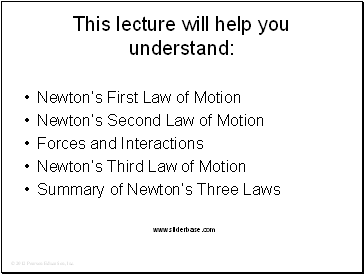Newton’s First Law of Motion

Newton’s Second Law of Motion

Forces and Interactions

Newton’s Third Law of Motion

Summary of Newton’s Three Laws

Slide 2Newton’s First Law of Motion

The law of inertia: (originating with Galileo)

Every object continues in a state of rest or of uniform speed in a straight line unless acted on by a nonzero force.

Slide 3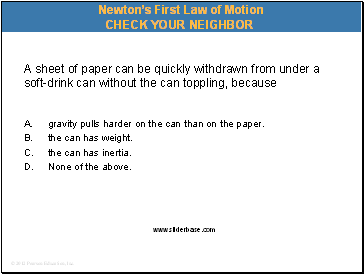A sheet of paper can be quickly withdrawn from under a soft-drink can without the can toppling, because

A. gravity pulls harder on the can than on the paper.

the can has weight.

the can has inertia.

None of the above.

Newton’s First Law of Motion

Slide 4A sheet of paper can be quickly withdrawn from under a soft-drink can without the can toppling, because

A. gravity pulls harder on the can than on the paper.

the can has weight.

the can has inertia.

None of the above.

Newton’s First Law of Motion

Slide 5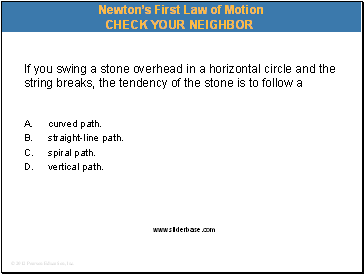If you swing a stone overhead in a horizontal circle and the string breaks, the tendency of the stone is to follow a

A. curved path.

straight-line path.

spiral path.

vertical path.

Newton’s First Law of Motion

Slide 6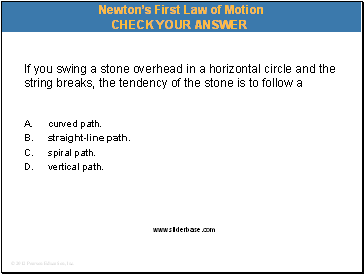If you swing a stone overhead in a horizontal circle and the string breaks, the tendency of the stone is to follow a

A. curved path.

straight-line path.

spiral path.

vertical path.

Newton’s First Law of Motion

Slide 7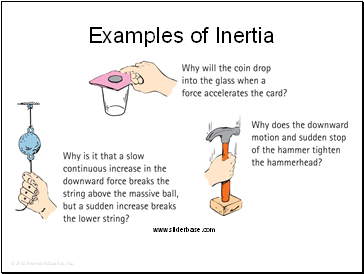Examples of Inertia

Slide 8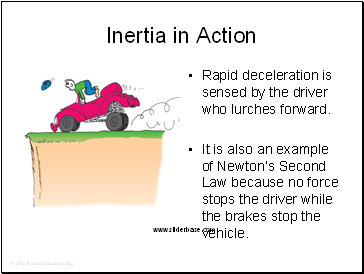Inertia in Action

Rapid deceleration is sensed by the driver who lurches forward.

It is also an example of Newton’s Second Law because no force stops the driver while the brakes stop the vehicle.

Slide 9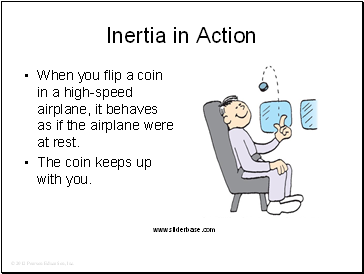Inertia in Action

When you flip a coin in a high-speed airplane, it behaves as if the airplane were at rest.

The coin keeps up with you.

Slide 10Inertia in Action

Can the bird drop down and catch the worm if the Earth moves at 30 km/s?

Go to page:
1  2  3  4  5  6  7  8  9